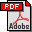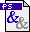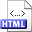Sorting multisets stably in minimum space Authors: Jyrki Katajainen and Tomi Pasanen Published in: Acta Informatica 31,4 (1994), 301-313 Full text:PDF (177 kB)PS (340 kB) DOI: 10.1007/BF01178508 Abstract: In a decision tree model, Ω(n log2 n − ∑i=1m ni log2 ni + n) is known to be a lower bound for sorting a multiset of size n containing m distinct elements, where the ith distinct element appears ni times. We present a minimum space algorithm that sorts stably a multiset in asymptotically optimal worst-case time. A Quicksort type approach is used, where at each recursive step the median is chosen as the partitioning element. To obtain a stable minimum space implemention, we develop linear-time in-place algorithms for the following problems, which have interest of their own:   Stable unpartitioning: Assume that an n-element array A is stably partitioned into two subarrays A0 and A1. The problem is to recover A from its constituents A0 and A1. The information available is the partitioning element used and a bit array of size n indicating whether an element of A0 or A1 was originally in the corresponding position of A.   Stable selection: The task is to find the kth smallest element in a multiset of n elements such that the relative order of identical elements is retained. Related:HTML (Conference paper) BibLATEX: ```@article{KP1994J, author = {Jyrki Katajainen and Tomi Pasanen}, title = {Sorting multisets stably in minimum space}, journaltitle = {Acta Informatica}, volume = {31}, number = {4}, year = {1994}, pages = {301--313}, } ```